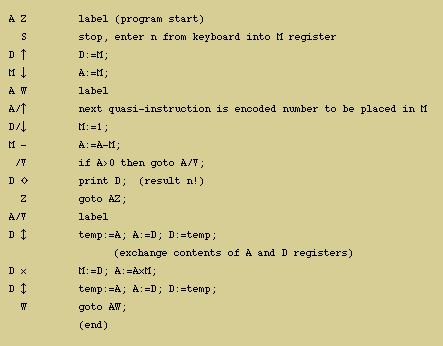# Olivetti Programma 101 Emulator

E.H. Dooijes, Computer Museum, University of Amsterdam, 1995

This program emulates on a PC most of the functionality of the Programma 101, a programmable desktop calculator marketed by Olivetti in the late '60's. The program runs on any PC (286 and up) with a VGA screen. The program together with its Turbo Pascal source code, is provided as a ZIP file.

The emulator uses the PC's standard arithmetic and 6-bytes real number representation. Though the P101's binary-coded decimal representation and fixed-point arithmetic are emulated by applying appropriate round-off (as determined by the decimal-indicator setting) to the intermediate results, it is not possible to obtain exactly the same answers (see for instance test program test3.prg, where the emulator's result is in fact more accurate than the P101's).

PROG101 only works in batch mode, i.e., it accepts program files as they used to be stored on magnetic cards. Program CON2SEQ translates a number into a sequence of quasi-operators, in which form it can be included in a PROG101 program. Likewise, coded numbers (as they are found in existing programs) can be translated back to decimal form using SEQ2CON. The emulator program files are text files in the same format as used in the program collections at the time available from Olivetti in printed form. Because not all of the machine's key symbols are in the ASCII set, we use the following alternatives:
 up arrow ^ down arrow \$ up-and-down arrow & diamond % star ! multiplication * division : square root #
The original symbols are shown in the next example program (also available as test4.prg).Each instruction takes the first three positions of a line. The first position is used for a register name (M,R,A-F); the second position is either empty or contains the / register-name modifier; the last position is for the operator. Opcodes may be entered in 'free format' in MAN101 only. Lines starting with # are regarded as comments.

The various registers are M, R, A, A/, B, B/, C, C/, D, D/, E, E/, F, F/. Like in the Olivetti handbooks, we use in this text (not in the programs) x as shorthand for X/.
In the P101, a and A are in fact the left and right parts of a single register containing 22 decimal places which is normally used for storing two 11-decimal numbers (plus decimal points and signs). In the emulator however a and A are completely separate registers. The same is true for the B, C, D, E and F registers.
In the P101, F, E and D are used for storing 24 instructions each as soon as the dedicated (invisible) instruction store's capacity of 48 is exceeded. In the emulator, on the contrary, the instructions are stored in a separate (invisible) buffer which will never overflow into the F, E and D registers.
These differences will normally have no influence on the performance of existing programs, unless the programmers have been using 'foul tricks'.
```+ (add)

instruction  phase_1 phase_2 rounded_result  complete_result

+               A + M         A              R
M +               A + M         A              R
R +      R-->M    A + M         A              R
A +      A-->M    A + M         A              R
a +      a-->M    A + M         A              R
B +      B-->M    A + M         A              R
b +      b-->M    A + M         A              R
C +      C-->M    A + M         A              R
c +      c-->M    A + M         A              R
D +      D-->M    A + M         A              R
d +      d-->M    A + M         A              R
E +      E-->M    A + M         A              R
e +      e-->M    A + M         A              R
F +      F-->M    A + M         A              R
f +      f-->M    A + M         A              R

- (subtract)
* (multiply)
similarly as +

: (divide)
computes in phase_2 the quotient A/M = q.
The result r (q rounded to n decimal places) is stored in A, and
(q-r)*M is placed in R (without being rounded). Hence with n=0,
R will contain A mod M.

# (square root)
A contains the rounded result, R remains undefined,
M contains twice the result.

& (abs)
A & sets the sign of the number in A to +, M and R remaining
unchanged.
```
```X ^     copies the contents of M into X. X is A|a|B|b|C|c|D|d|E|e|F|f.
X \$     copies the contents of X into A. X is M|B|b|C|c|D|d|E|e|F|f.
X &     swaps the contents of X and A.   X is M|B|b|C|c|D|d|E|e|F|f.
/&     copies the decimal part of A to M.
```
```X/      clears X.                        X is B|b|C|c|D|d|E|e|F|f.
X %     prints X
/%     prints an empty line.
S     prompts the user (by >>) to enter either a number or a
directive:
q to quit the program or (in MAN101 only):
? help
r toggle register display mode (default: display enabled)
d choose decimal indicator position (default 4).
R S     swaps the contents of registers R and D.
```
1. It is possible, for instance by specifying the wrong start address, to force the program into a loop which (in the present version) only can be broken by resetting the PC.
2. In most error conditions the emulator will report what is going wrong. The original P101 used red and green lights as status indicators; also the keyboard would be blocked at certain errors.
3. Input data and results (printed on paper by the P101) are written to a textfile "results".
4. PROG101 shows at every input and output operation first the number of the current instruction.
5. PROG101 can be terminated by typing "q" (instead of a number) after the >> prompt.

rev February 27, 2017